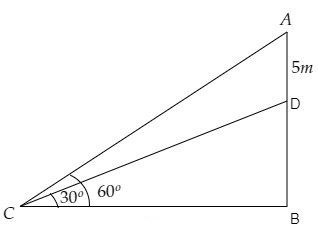# A vertical tower stands on a horizontal plane and is surmounted by a vertical flag-staff of height 5 metres. At a point on the plane, the angles of elevation of the bottom and the top of the flag-staff are respectively $30^{\circ}$ and $60^{\circ}$. Find the height of the tower.

Given:

A vertical tower stands on a horizontal plane and is surmounted by a vertical flag-staff of height 5 metres. At a point on the plane, the angles of elevation of the bottom and the top of the flag-staff are respectively $30^{\circ}$ and $60^{\circ}$.

To do:

We have to find the height of the tower.

Solution:Let $DB$ be the tower and $AD$ be the length of the flag-staff.

Let point $C$ be the point of observation.

From the figure,

$\mathrm{AD}=5 \mathrm{~m}, \angle \mathrm{DCB}=30^{\circ}, \angle \mathrm{ACB}=60^{\circ}$

Let the height of the tower be $\mathrm{DB}=h \mathrm{~m}$ and the distance between the point $C$ and the foot of the tower be $\mathrm{BC}=x \mathrm{~m}$.

We know that,

$\tan \theta=\frac{\text { Opposite }}{\text { Adjacent }}$

$=\frac{\text { DB }}{BC}$

$\Rightarrow \tan 30^{\circ}=\frac{h}{x}$

$\Rightarrow \frac{1}{\sqrt3}=\frac{h}{x}$

$\Rightarrow x=h(\sqrt3) \mathrm{~m}$

$\Rightarrow x=\sqrt3 h \mathrm{~m}$...........(i)

Similarly,

$\tan \theta=\frac{\text { Opposite }}{\text { Adjacent }}$

$=\frac{\text { AB }}{BC}$

$\Rightarrow \tan 60^{\circ}=\frac{5+h}{x}$

$\Rightarrow \sqrt3=\frac{5+h}{x}$

$\Rightarrow x\sqrt3=5+h \mathrm{~m}$

$\Rightarrow (\sqrt3 h)\sqrt3=5+h \mathrm{~m}$           [From (i)]

$\Rightarrow 3h=5+h \mathrm{~m}$

$\Rightarrow 3h-h=5 \mathrm{~m}$

$\Rightarrow h=\frac{5}{2}=2.5 \mathrm{~m}$

Therefore, the height of the tower is $2.5 \mathrm{~m}$.

Updated on: 10-Oct-2022

39 Views